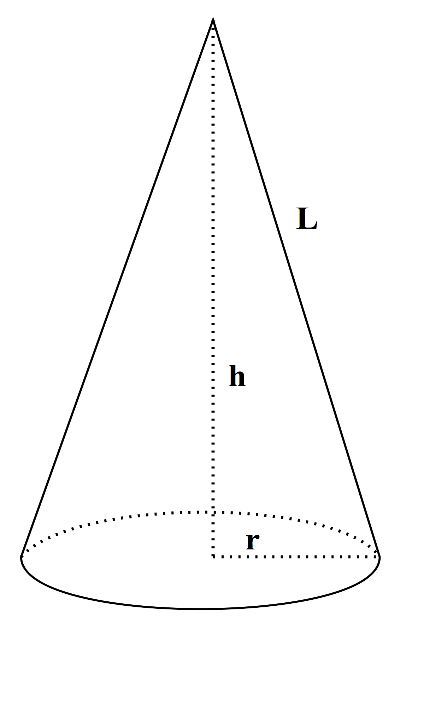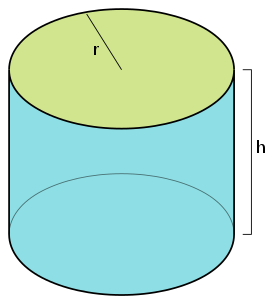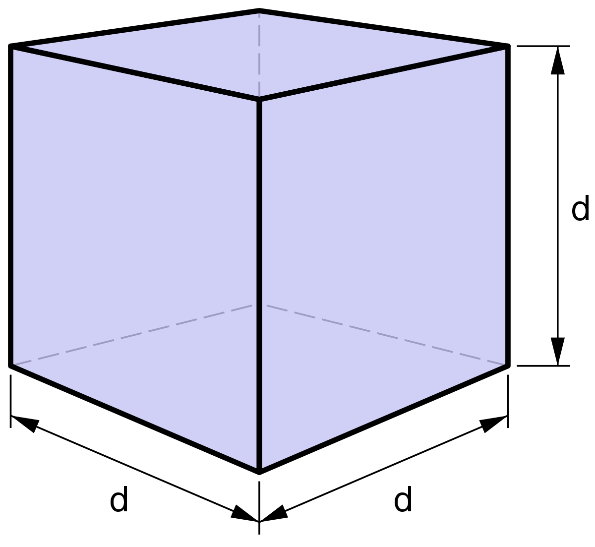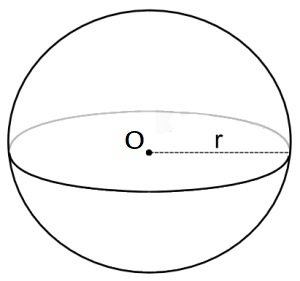## Surface Area calculator for 3D shapes

#### Surface Area Calculator

Result:

 Surface area of Cone: 0

Result:

 Surface area of Cylinder: 0

Result:

 Surface area of Cube: 0

Result:

 Surface area of Sphere: 0

Result:

 Surface area of Rectangluar Prism: 0

Result:

 Surface area of Triangular Prism: 0

### What is Surface Area?

Area defines the region covered by a two-dimensional flat surface. The area covered by a three-dimensional body by its outer surface is known as the surface area. The surface area of a three-dimensional object refers to the area including the base and the curved part. The total surface area is the sum of the area covered by the base and the area covered by the curved part (called the lateral surface area).

### Surface Area Formulas

Below are the formulas to calculate the surface areas of three-dimensional shapes.

#### Cone

In three-dimensional geometry, a cone is a shape which becomes narrower smoothly from a flat base (sometimes, circular) to a point called the apex or vertex. Given below is the figure of a cone.In this figure, the base of the cone is a circle of radius r. The distance from the centre of the base to the vertex of the cone is called the height h of the cone. The distance from the vertex to any point on the circumference of the base is called the slant height of the cone indicated by L.

The slant height can be calculated as $$L \;= \; \sqrt{(r^2+h^2)}$$

The surface area of a cone can be found using the formula $$πr^2 \;+ \;πr \, \sqrt{(r^2+h^2)} \;square \;units$$

#### Cylinder

A cylinder is a three-dimensional shape which consists of two parallel circular bases separated by a distance. The circular cases are joined by a curved surface at a fixed distance from their centres. The centres of the two circular bases are connected by a line which is called the axis of the cylinder. The figure below shows a cylinder.In this figure, the two circular bases have the same radius r. They are separated by a distance h. This is known as the height of the cylinder.

The surface area of the cylinder is calculated as $$2πr \,(r+h) \;square \;units$$

#### Cube

A cube is a three-dimensional solid body with six square sides (or faces), twelve edges and eight vertices. Three of the sides meet at each vertex. The cube is a special case of a cuboid (or rectangular prism) where the length, breadth and height are equal. The figure below shows a cube of side ‘d’ units.The surface area of a cube is $$6d^2 \;square \;units$$

#### Rectangular prism

A rectangular prism is a three-dimensional figure with six sides, eight vertices and twelve edges. It has two parallel bases. It is also called a cuboid. Each of the six sides are rectangular in shape. The figure below shows a rectangular prism.The formula for the surface area of a rectangular prism is $$2(lb+bh+lh) \;square \;units,$$ where l, b and h denote the length, breadth and height of the rectangular prism as shown in the figure.

#### Sphere

A sphere is a round solid in three-dimensional space. Like a circle in a two-dimensional space, a sphere is mathematically defined as the set of points which are the same distance ‘r’ from a specific point in three-dimensional space. This distance ‘r’ is called the radius of the sphere, and the given point is called its centre. The figure below shows a sphere of radius r and centre O.The formula for the surface area of a sphere is $${4\over3}πr^2 \;square \;units$$

#### Triangular prism

A triangular prism is a polyhedron (or three-dimensional shape) having five sides, six vertices and nine edges. It consists of two triangular bases, and three rectangular sides. The edges of the prism join the corresponding sides.The figure above shows a triangular prism where the length of the rectangular side is L, b is the length of the triangular base and h is the height of the base. The surface area of the prism is the sum of the lateral surface area and twice the area of the triangular base.

If a, b, and c indicate the sides of the triangle, the area is $$\sqrt{s(s-a)(s-b)(s-c)} \;square \;units$$ where 's' is the semi-perimeter, $$s \; = \; {{a+b+c}\over 2}$$

The lateral surface area is $$(a+b+c)L \;square \;units$$

Hence, the total surface area is $$2 \sqrt{(s(s-a)(s-b)(s-c))} \;+ \;(a+b+c)L \;square \;units.$$

If the height of the triangular base is known, then the area of the triangular base is $${1\over2} \,bh \;square \;units.$$ In that case, the total surface area is $$bh+(a+b+c)L \;square \;units.$$

### Areas of application

Like volume, surface area formulas are also used quite frequently in daily life.

• When we want to know how much paint is required to paint a wall, or how much wallpaper is required to cover it completely, the surface area formula is used.
• We need to know the surface area of a cylinder to find out how much paint is required for painting a fuel tank.
• The amount of cloth needed to make a piece of clothing is determined using the surface area.
• A toy manufacturer who needs to find out how much plastic he needs to make balls would first need to know the surface area of the ball he is making.